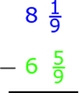# Peter's calculation

Peter wrote the following: 7 1/4 - 3 3/4 = 4 2/4 = 4 1/2 . Is Peter’s calculation correct? Using words (math vocabulary) and numbers explain why he is correct or incorrect.

x=Did you find an error or inaccuracy? Feel free to write us. Thank you!

Showing 1 comment:Dr Math
Can't be subtracted whole parts and fractions part in opposite direction.

7 1/4 - 3 3/4 = whole (7-3) and fraction part (3-1)/4 = 4 and fraction part 2/4 = 4 1/2Tips to related online calculators
Need help to calculate sum, simplify or multiply fractions? Try our fraction calculator.